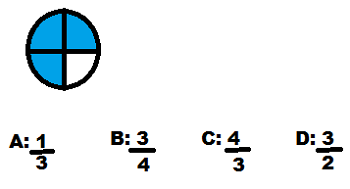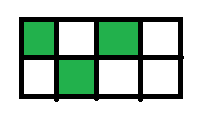# Fractions: Lesson for Kids

Instructions:

question 1 of 3

### What fraction of the circle is shaded blue?Create Your Account To Take This Quiz

As a member, you'll also get unlimited access to over 79,000 lessons in math, English, science, history, and more. Plus, get practice tests, quizzes, and personalized coaching to help you succeed.

Try it risk-free for 30 days. Cancel anytime

### 1. Look at the rectangle. If you were to write a fraction, what would be the denominator?### 2. If you were to write a fraction for the parts of this rectangle that are white, what would your numerator be?Create your account to access this entire worksheet
Quizzes, practice exams & worksheets
Certificate of Completion
Create an account to get started

Take this quiz and print this worksheet to see how well you understand fractions by solving practice problems. The questions are multiple-choice and ask you to look at pictures then choose the letter or number that represents the correct answer.

## Quiz & Worksheet Goals

On this quiz and worksheet, you will be asked to:

• Find the fractions of shaded sections
• Choose the correct denominator of a fraction
• Select the correct numerator of a fraction

## Skills Practiced

Use this quiz and worksheet to practice the following skills:

• Problem solving - use acquired knowledge to solve fraction practice problems
• Distinguishing differences - compare and contrast topics from the lesson, such as numerator and denominator

• Definition of a fraction